Home MATHEMATICS TOPIC 2: FRACTIONS ~ MATHEMATICS FORM 1

# TOPIC 2: FRACTIONS ~ MATHEMATICS FORM 1

4726
143## TOPIC 2: FRACTIONS

A fraction is a number which is expressed in the form of​​ a/b​​ where​​ a –​​ is the top number called numerator and​​ b– is the bottom number called denominator.

Proper, Improper and Mixed Numbers

#### A Fraction

Describe a fraction

A fraction is a number which is expressed in the form of​​ a/b​​ where​​ a –​​ is the top number called numerator and​​ b– is the bottom number called denominator.

Consider the diagram below

The shaded part in the diagram above is 1 out of 8, hence mathematically it is written as 1/8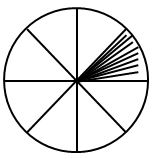Example 1

(a) 3 out of 5 ( three-fifths) = 3/5

Example 2

(b) 7 0ut of 8 ( i.e seven-eighths) = 7/8

Example 3

5/12=(5 X 3)/(12 x 3) =15/36

3/8 =(3 x 2)/(8 X 2) = 6/16

Dividing the numerator and denominator by the same number (This method is used to simplify the fraction)

Difference between Proper, Improper Fractions and Mixed Numbers

Distinguish proper, improper fractions and mixed numbers

Proper fractionis a fraction in which the numerator is less than denominator

Example 4

4/5, 1/2, 11/13

Improper fraction​​ -is a fraction whose numerator is greater than the denominator

Example 5

12/7, 4/3, 65/56

Mixed fraction –is a fraction which consist of a whole number and a proper fraction

Example 6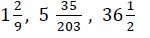(a) To convert mixed fractions into improper fractions, use the formula below

(b)To convert improper fractions into mixed fractions, divide the numerator by the denominator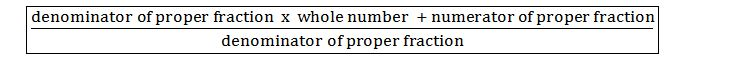Example 7

Convert the following mixed numbers into improper fractions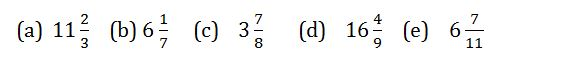#### Comparison of Fractions

In order to find which fraction is greater than the other, put them over a common denominator, and then the greater fraction is the one with greater numerator.

TOPIC 7: GEOMETRIC AND TRANSFORMATIONS ~ MATHEMATICS FORM 2

A Fraction to its Lowest Terms

Simplify a fraction to its lowest terms

Example 8

For the pair of fractions below, find which is greater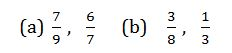Solution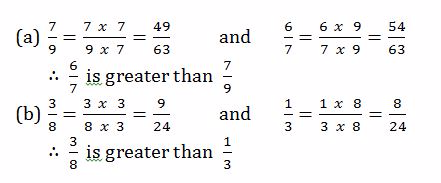#### Equivalent Fractions

Identify equivalent fractions​​

Equivalent Fraction – Are equal fractions written with different denominators

They are obtained by two methods

<!– [if !supportLists]–>(a)​​ <!–[endif]–>Multiplying the numerator and denominator by the same number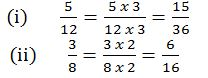<!– [if !supportLists]–>(a)​​ <!–[endif]–>Dividing the numerator and denominator by the same number (This method is used to simplify the fraction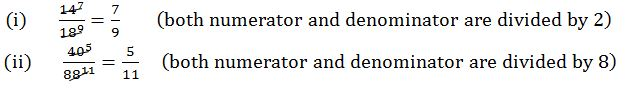NOTE: The fraction which cannot be simplified more is said to be in its lowest form

Example 9

Simplify the following fractions to their lowest terms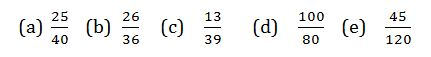Solution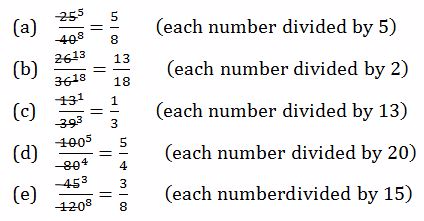#### Fractions in Order of Size

Arrange fractions in order of size

Example 10

Arrange in order of size, starting with the smallest, the fraction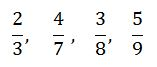Solution

Put them over the same denominator, that is find the L.C.M of 3, 7, 8 and 9

### Operations and Fractions

Operations on fractions involves​​

2. Subtraction,

3. Multiplication ​​

4. Division

Addition and subtraction of fractions is done by putting both fractions under the same denominator and then add or subtract

Multiplication of fractions is done by multiplying the numerator of the first fraction with the numerator of the second fraction, and the denominator of the first fraction with the denominator the second fraction.

For mixed fractions, convert them first into improper fractions and then multiply

Division of fractions is done by taking the first fraction and then multiply with the reciprocal of the second fraction

For mixed fractions, convert them first into improper fractions and then divide

TOPIC 5: APPROXIMATIONS | MATHEMATICS FORM 1

Example 11

Find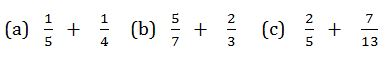Solution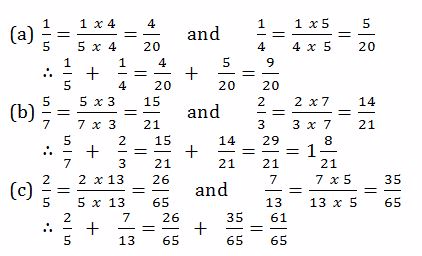#### Subtraction of Fractions

Subtract fractions

Example 12

Evaluate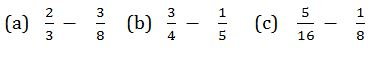Solution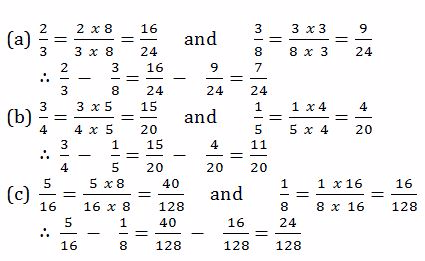#### Multiplication of Fractions

Multiply fractions​​

Example 13